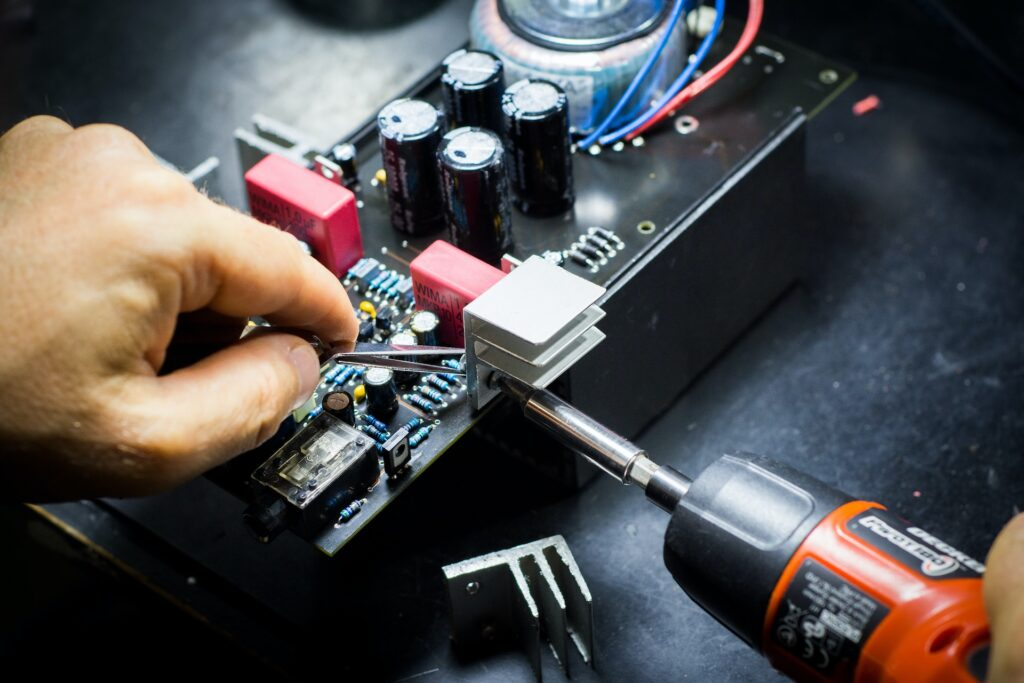# Resistor Types and Applications

Introduction:

Resistors are one of the fundamental components in electronic circuits that limit the flow of current and voltage in a circuit. There are various types of resistors available in the market, and each has its unique properties and uses. In this article, we will discuss the different types of resistors and their uses.

1. Carbon Composition Resistors:

Carbon composition resistors are the most commonly used resistors in electronic circuits. They are made of a mixture of carbon particles and a binder material. Carbon composition resistors have a high tolerance and low noise level, making them suitable for use in low-frequency circuits.

1. Film Resistors:

Film resistors are made by depositing a thin layer of resistive material on a ceramic or fiberglass substrate. They are more precise and stable than carbon composition resistors and are used in applications that require high precision and stability, such as in audio and video equipment.

1. Wire-wound Resistors:

Wire-wound resistors are made by winding a wire around a ceramic core. They have a higher power rating and lower temperature coefficient than other types of resistors, making them ideal for high-power applications such as power supplies.

1. Variable Resistors:

Variable resistors, also known as potentiometers or rheostats, allow the resistance to be changed by rotating a knob or moving a slider. They are used in circuits where it is necessary to adjust the resistance, such as in volume control or tuning circuits.

1. Thermistors:

Thermistors are resistors whose resistance changes with temperature. They are used in temperature sensing circuits, and their resistance decreases with an increase in temperature (Negative Temperature Coefficient) or increases with an increase in temperature (Positive Temperature Coefficient).

1. Light Dependent Resistors:

Light-dependent resistors (LDRs) are resistors whose resistance changes with the intensity of light. They are used in light sensing circuits, such as automatic streetlights and cameras.

Conclusion:

In conclusion, resistors are essential components in electronic circuits that limit the flow of current and voltage. The type of resistor used depends on the application, and each type has its unique properties and uses. Carbon composition resistors, film resistors, wire-wound resistors, variable resistors, thermistors, and light-dependent resistors are some of the commonly used resistors in electronic circuits. It is essential to choose the appropriate resistor for the circuit to ensure optimal performance and reliability.